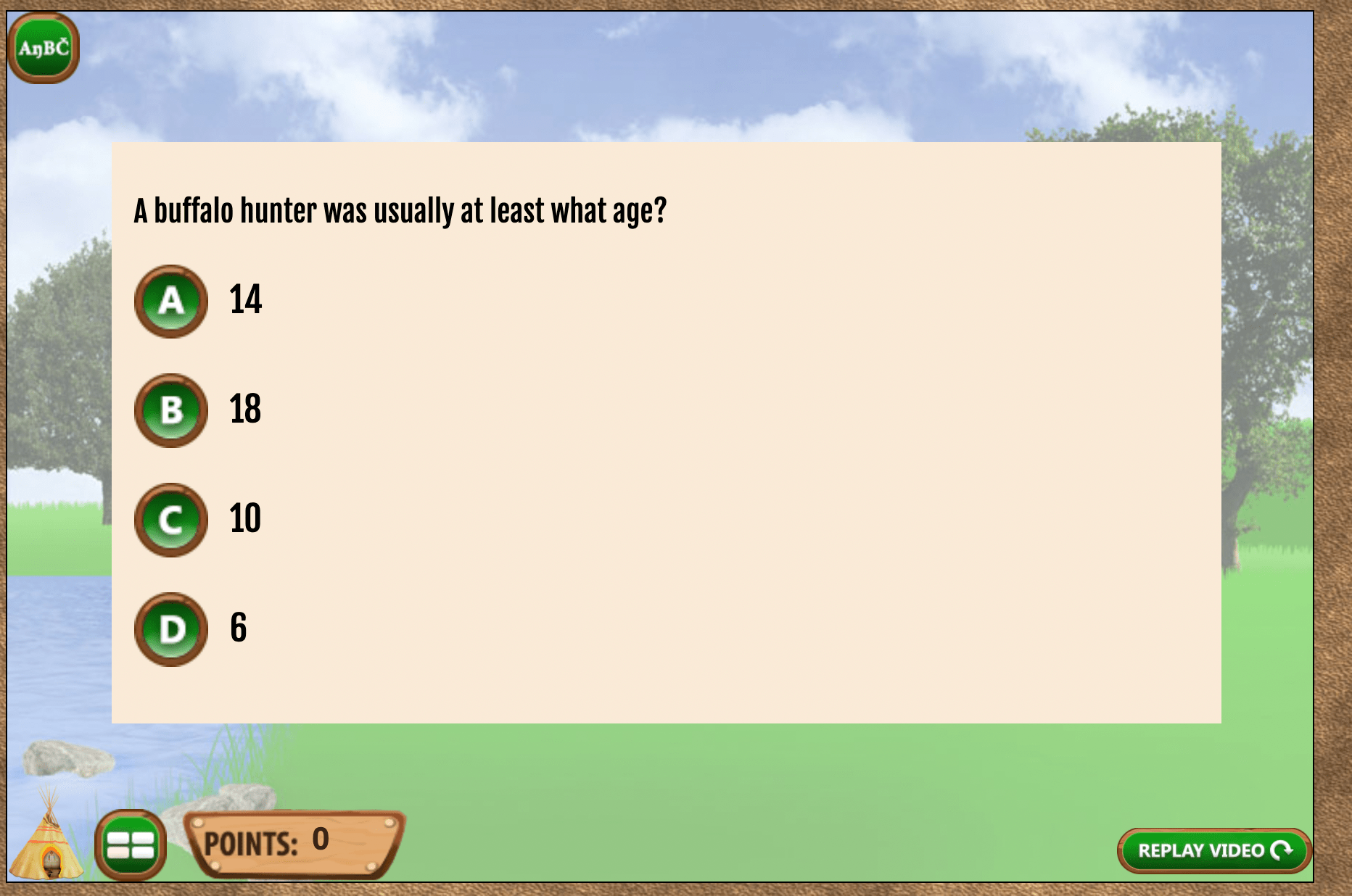# Archives# Math Assessment and Lakota Culture

## Standard

CCSS.MATH.CONTENT.8.EE.A.1
Know and apply the properties of integer exponents to generate equivalent numerical expressions. For example, 32 × 3-5 = 3-3 = 1/33 = 1/27.

CCSS.MATH.CONTENT.5.NBT.B.7
Add, subtract, multiply, and divide decimals to hundredths,

NOTE: Although the examples in this assignment primarily use scientific notation, it could be easily modified to include any area of mathematics above the fourth-grade level.

##TIME

120 minutes – including time to play game and create problems and activities.

##TECHNOLOGY REQUIRED

A projector and computer in class or a computer, phone or tablet at home is required to watch the videos, see the Google slides and play the Making Camp Lakota game. It is not required but strongly recommended that students have access to Google apps or Office 365 to edit and store their notes.

##LESSON SUMMARY

This is the second in a multi-lesson unit designed to assess student mathematics proficiency by playing games that teach about Indigenous cultures that have embedded math problems. It also requires students to create their own math activities and math problems.

#LESSON

### Introduce today’s lesson with slide presentation

This lesson assumes your class did the previous lesson, Math Assessment and Ojibwe culture, where they were introduced to the purposed of the unit and (recommended) created a Google slides or doc file where they are taking notes.

To introduce today’s lesson with Making Camp Lakota, use this PowerPoint on Math Assessment and Lakota culture, with examples of new, more difficult problems using decimals and scientific notation.

### Play a Game

The Making Camp Lakota game teaches Lakota culture and division with single-digit divisors. Even older students should enjoy the game play aspects and the videos on Lakota history and culture. Middle school students should breeze through the math problems. These are recorded in the database for teachers to review student progress.

Each game in the series in this unit is gradually more difficult math problems.

### Students create their own, grade-level math problems

The slides presentation instructs students, for each math activity, to create an example that could be used at their grade level. Most of the examples in this presentation are using scientific notation, but students should be instructed that they can use any math problems beyond simple division. That could be fractions, decimals or even long-division. Teachers can modify the slides at the end of the presentation to require a specific topic, for example, adding fractions without a common denominator.

## ASSESSMENT

Making Camp Lakota teacher reports are available for assessing student answers in Data and Reports. Students also write their own problems and answers that the teacher can use for assessing abilities at application and creation levels of Bloom’s taxonomy.

## Related Lessons

For students who need an introduction to Google apps and Google doc, the lesson Google Apps Basics for Hamsters is recommended. (You don’t need an actual hamster.)

## Individualization

This lesson is appropriate for students whose math is from fourth- through eighth-grade level. The mathematics in the game is at the fourth-grade level but student assignments can be as simple as long division or as complex as multi-step equations with negative exponents.# Math Assessment and Ojibwe Culture

## Standard

CCSS.MATH.CONTENT.8.EE.A.1
Know and apply the properties of integer exponents to generate equivalent numerical expressions. For example, 32 × 3-5 = 3-3 = 1/33 = 1/27.

NOTE: Although the examples in this assignment use exponents, it could be easily modified to include any area of mathematics above the fourth-grade level.

##TIME

120 minutes – including time to play game and create problems and activities.

##TECHNOLOGY REQUIRED

A projector and computer in class or a computer, phone or tablet at home is required to watch the videos, see the Google slides and play the Making Camp Premium game. It is not required but strongly recommended that students have access to Google apps or Office 365 to edit and store their notes.

##LESSON SUMMARY

This is the first in a multi-lesson unit designed to assess student mathematics proficiency by playing games that teach about Indigenous cultures that have embedded math problems. It also requires students to create their own math activities and math problems.

#LESSON

## Introduce lesson with slide presentation

The objectives, link to the first game and sample questions can all be found in this Slides presentation. The examples here use Exponent Rules and creating a math problem that can be solved with an equation using exponents. However, teachers are free to download the video and modify to fit their own class.

## Watch a video

Even students who are Native American themselves sometimes need to be reminded when asked to apply mathematics to needs of Indigenous communities. This seven-minute video on Tribal Epidemiology Centers is a great example to get students thinking.

## Play a Game

The Making Camp Premium game teaches Ojibwe culture, multiplication and division. Even older students should enjoy the game play aspects and the videos on Ojibwe history and culture. Middle school students should breeze through the math problems. These are recorded in the database for teachers to review student progress.

## Students create their own, grade-level math problems

The slides presentation instructs students, for each math activity, to create an example that could be used at their grade level. The two examples in the presentation use exponents but students are told they can use any mathematics above multiplication and division. That could be fractions, decimals or even long-division. Teachers can modify the slides at the end of the presentation to require a specific topic, for example, adding fractions without a common denominator.

## ASSESSMENT

Making Camp Premium teacher reports are available for assessing student answers in Data and Reports. Students also write their own problems and answers that the teacher can use for assessing abilities at application and creation levels of Bloom’s taxonomy.

## Related Lessons

For students who need an introduction to Google apps and Google doc, the lesson Google Apps Basics for Hamsters is recommended. (You don’t need an actual hamster.)